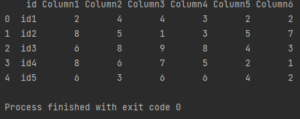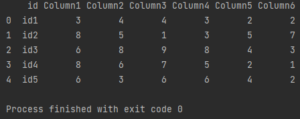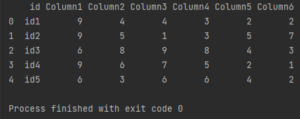Pandas How To Uncategorized How to replace values in a column

# How to replace values in a column

Working with data is not easy. Not only you have to prepare, clean and interpret them, you also often have to correct the data. This is a tutorial on how to replace values in a column in a Pandas dataframe.

First of all, I’d like to reassure you. Correcting values in dataframe columns is easy in Pandas. The easy part is that Pandas offers a dedicated replace function that allows you to replace data.

This post is really a description of the use cases of the replace function in Pandas. I’ll walk you through some different examples of using the replace function so you can learn how to replace data in Pandas using the replace function.

## How to replace values in a column

Here is my sample dataframe that I use to replace the data in this post.

```import pandas as pd

my_df = pd.DataFrame({'id':['id1','id2','id3','id4','id5'],
'Column1': ['2','7','6','7','6'],
'Column2': ['4','5','8','6','3'],
'Column3': ['4','1','9','7','6'],
'Column4': ['3','3', '8','5',6],
'Column5': ['2','5','4','2','4'],
'Column6': ['2','7','3','1','2']})

print(my_df)

```

In this example, I want to replace one of the values in the first column.

```import pandas as pd

my_df = pd.DataFrame({'id':['id1','id2','id3','id4','id5'],
'Column1': ['2','7','6','7','6'],
'Column2': ['4','5','8','6','3'],
'Column3': ['4','1','9','7','6'],
'Column4': ['3','3', '8','5',6],
'Column5': ['2','5','4','2','4'],
'Column6': ['2','7','3','1','2']})

my_df['Column1'] = my_df['Column1'].replace('7','8')

print(my_df)

```As you can see the value changed in two rows.

## How to replace multiple values in a column

The replace function also allows you to replace multiple values in a column in a simple way. To replace multiple values with a single command, just list them in square brackets.

```import pandas as pd

my_df = pd.DataFrame({'id':['id1','id2','id3','id4','id5'],
'Column1': ['2','7','6','7','6'],
'Column2': ['4','5','8','6','3'],
'Column3': ['4','1','9','7','6'],
'Column4': ['3','3', '8','5',6],
'Column5': ['2','5','4','2','4'],
'Column6': ['2','7','3','1','2']})

my_df['Column1'] = my_df['Column1'].replace(['7','2'],['8','3'])

print(my_df)

```Be careful not to make a mistake. In the first square brackets, list the values you want to change. In the second bracket, the ones you want to change to. The first value in the first bracket will be replaced with the first value in the second bracket, and so on.

## How to replace multiple values in a column to one value

It is also possible to convert multiple values in a column to a single value. To do this, you enter the old values in square brackets, and the new value simply after a comma.

```import pandas as pd

my_df = pd.DataFrame({'id':['id1','id2','id3','id4','id5'],
'Column1': ['2','7','6','7','6'],
'Column2': ['4','5','8','6','3'],
'Column3': ['4','1','9','7','6'],
'Column4': ['3','3', '8','5',6],
'Column5': ['2','5','4','2','4'],
'Column6': ['2','7','3','1','2']})

my_df['Column1'] = my_df['Column1'].replace(['7','2'],'9')

print(my_df)

```Here are the most common cases of replacing a value in a dataframe column in Pandas. I hope you solved your problem in Pandas. Also check other entries on my website.

Tags:

## How to print a full dataframeHow to print a full dataframe

To print a full dataframe in Python, you can use the pd.set_option() function from the Pandas library to set the maximum number of columns and rows to be displayed. Here’s

## How to remove duplicate rows based on multiple columnsHow to remove duplicate rows based on multiple columns

You can remove duplicate rows based on multiple columns in Pandas by using the drop_duplicates method. Here is an example of how you could remove duplicate rows based on multiple

## How to remove outliers in PandasHow to remove outliers in Pandas

There are several methods to remove outliers in Pandas, here are a few commonly used techniques: Z-Score Method: Calculate the z-score of each data point, and remove those with a